# Kenya Certificate Of Secondary Education(KCSE 2013) Mathematics Alt A Paper 1 with Marking Scheme

SECTION I (50mks)
Answer all the questions in this section in the spaces provided.

1. Evaluate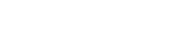( 2 marks)
2. The production of milk, in litres, of 14 cows on a certain day was recorded as follows:
22,26,15,19,20,16,27,15,19,22,21,20,22 and 28.
Determine:
1. the mode (1 mark)
2. the median (2 marks)
3. Use logarithms, correct to 4 decimal places, to evaluate:(4 marks)
4. Simplify the expression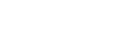(3 marks)
5. A wholesaler sold a radio to a retailer making a profit of 20%. The retailer later sold the radio for Ksh 1,560 making a profit of 30%. Calculate the amount of money the wholesaler had paid for the radio. (3 marks)
6. A point P on the line AB shown below is such that AP = 2/7 AB. By construction, locate P. (3 marks)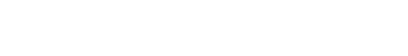7. Chelimo's clock loses 15 seconds every hour. She sets the correct time on the clock at 0700h on a Monday. Determine the time shown on the clock when the correct time was 1900h on Wednesday the same week. (3 marks)
8. Given that sin(x + 20)° = - 0.7660 find x, to the nearest degree, for 0° ≤ x ≤ 360° . (3 marks)
9. A number m is formed by writing all the prime numbers between 0 and 10 in an ascending order. Another number n is formed by writing all the square numbers between 0 and 10 in descending order.
1. Find m-n (2 marks)
2. Express (m-n) as a product of its prime factors (1 mark)
3. The figure below shows a net of a solid. (Measurements are in centimetres).
10. The figure below shows a net of a solid. (Measurements are in centimetres).Below is a part of the sketch of the solid whose net is shown above.Complete the sketch of the solid, showing the hidden edges with broken lines. (3 marks)
11. The interior angles of an octagon are 2x, ½x,(x + 40)°, 110°, 135°, 160°, (2x + 10)° and 185°. Find the value of x. (2 marks)
12. A straight line passes through the points (-2,1) and (6,3).
Find:
1. the equation of the line in the form y = mx + c; (2 marks)
2. the gradient of a line perpendicular to the line in (a). (1 mark)
13. A triangle ABC is such that AP = 5cm, BC = 6cm and AC = 7cm.
1. Calculate the size of angle ACB, correct to 2 decimal places (2 marks)
2. A perpendicular drawn from A meets BC at N. Calculate the length AN correct to one decimal place (2 marks)
14. A cylindrical pipe 2½ metres long has an internal diameter of 21 millimetres and an external diameter of 35 millimetres. The density of the material that makes the pipe is 1.25 g/cm3. Calculate the mass of the pipe in kilograms. (Take π = 22/7) (4 marks)
15. The figure below represents a pentagonal prism of length 12cm. The cross-section is a regular pentagon of side 5cm.Calculate the surface area of the prism correct to 4 significant figures. (4 marks)
16. Given the inequalities x-5 <= 3x-8 < 2x-3
1. Solve the inequalities; (2 marks)
2. represent the solution on a number line

SECTION II (50 marks)
Answer only five questions in this section in the spaces provided

1. A farmer had 540 bags of maize each having a mass of 112kg. After drying the maize, the mass decreased in the ratio 15:16.
1. Calculate the total mass lost after the maize was dried. (3 marks)
2. A trader bought and repacked the dried maize in 90kg bags. He transported the maize in a lorry which could carry a maximum of 120 bags per trip.
1. Determine the number of trips the lorry made. (3 marks)
3. The buying price of a 90 kg bag of maize was Ksh 1500. The trader paid Ksh 2500 per trip to transport the maize to the market. He sold the maize and made a profit of 26 %. Calculate the selling price of each bag of the maize. (4 marks)
2.
1. Solve the equation,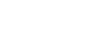(4 marks)
2. The length of a floor of a rectangular hall is 9 m more than its width. The area of the floor is 136 m2.
1. Calculate the perimeter of the floor (4 marks)
2. A rectangular carpet is placed on the floor of the hall leaving an area of 64 m2. If the length of the carpet is twice its width, determine the width of the carpet (2 marks)
3. A trader bought 2 cows and 9 goats for a total of Ksh 98,200. If she had bought 3 cows and 4 goats, she would have spent Ksh 2,200 less.
1. Form two equations to represent the above information. (2 marks)
2. Use matrix method to determing the cost of a cow and that of a goat. (4 marks)
3. The trader later sold the animal she had bought making a profit of 30% per cow and 40% per goat.
1. Calculate the total amount of money she received. (2 marks)
2. Determine, correct to 4 significant figures, the percentage profit the trader made from the sale of the animals. (2 marks)
4. Two towns, A and B are 80km apart. Juma started cycling from town A to town B at 10:00 am at an average speed of 40 km/h. Mutuku started his journey from town B to town A at 10:30 am and travelled by car at an average speed of 60 km/h.
1. Calculate:
1. the distance from town A when Juma and Mutuku met; (5 marks)
2. the time of the day when the two met (2 marks)
2. Kamau started cycling from town A to town B at 10:21 am. He met Mutuku at the same time as Juma did. Determine Kamau's average speed. (3 marks)
5. The displacement, s metres, of a moving particle from a point O, after t seconds is given by, s= t3 - 5t2 + 3t + 10
1. Find s when t=2 (2 marks)
2. Determine:
1. the velocity of the particle when t = 5 seconds; (3 marks)
2. the value of t when the particle is momentarily at rest. (3 marks)
3. Find the time, when the velocity of the particle is maximum (2 marks)
6. In the figure below, OABC is a trapezium. AB is parallel to OC and OC = 5AB. D is a point on OC such that OD:DC = 3:2.1. Given that OA = p and AB = q, express in terms of p and q:
1. OB; (1 mark)
3. CB. (2 marks)
2. Lines OB and AD intersect at point X such that AX = kAD and OX = rOB, where k and r are scalars. Determine the values of k and r (5 marks)
7.
1. On the grid provided, draw the square whose vertices are A(6,-2), B(7, -2), C(7, -1) and D(6,-1). (1 mark)
2. On the same grid, draw:
1. A'B'C'D', the image of ABCD, under an enlargement scale factor 3, centre (9,-4);
2. A"B"C"D", the image of A'B'C'D' under a reflection in the line x = 0; (2 marks)
3. A"'B"'C"'D"', the image of A"B"C"D" under a rotation of +90 about (0,0) (2 marks)
3. Describe a single transformation that maps A'B'C'D' onto A'"B"'C"'D"' (2 marks)
8. The figure below represents a cone of height 12cm and base radius of 9cm from which a similar smaller cone is removed, leaving a conical hole of height 4cm.1. Calculate:
1. the base radius of the conical hole; (2 marks)
2. the volume, in terms of π, of the smaller cone that was removed (2 marks)
2.
1. Determine the slant height of the original cone. (1 mark)
2. Calculate, in terms of π, the surface are of the remaining solid after the smaller cone is removed. (5 marks)## MARKING SCHEME

1  36  - -108
-12     -27
=-3-4
=-7
M1
A1
2

2
1. Mode
= 22
2. Median
15, 15, 16, 19, 19, 20, 20, 21, 22, 22, 22, 26, 27, 28
median = 20+21
2
= 20.5
B1
M1
A1
3

3
 No Log 1.7940.0381.243 0.25382.57982.83360.0945 0.3799 2.7391 ÷ 31.5797

M1
M1
M1
A1
4

all log
+ and operations
÷ 3
4 (4m + 3n)(4m - 3n)
(4m + 3n)(m-n)
= 4m - 3n
m -n
M1
M1
A1
factorizing numerator
factorizing denominator
5 Retailer
130% →1560
100% → 1560 x 100
130
= 1200
Wholesaler
120% → 1200
100% → 1200 x 100
120
= 1000
M1
M1
A1
3

6B1
B1
B1
3
construction of equal parts on AC
draw DP/CB such that AP= 2/7 AB
locating point P
7 From 0700 h Monday to 1900 h Wednesday
= 24 x 2 + 12 h
= 60 h
Time lost = 60 x 15 = 900 sec
= 15 min
Time shown on clock:
1900 h -15 min = 1845 h
M1
M1
A1
3

8 x + 20 = 230° or x + 20 = 310°
x = 210°
or
x = 290°
B1
B1
B1
3
for 230° or 310°
9
1. 2357 - 941 = 1416
2. 1416 = 23 x 3 x 59
B1
B1
B1
3
for 2357 and 941 ✓
for 1416
10B1
B1
B1
3
lines AF, ED equal and parallel to BC
lines AB, FC equal and parrallel or B1 lines AE and FD equal and parallel or lines CD, EB equal and parallel.
completing the solid showing dotted lines.
11 2x + ½x + x + 40 + 110 + 135 + 160 + 2x + 10 + 185
= 1080
11/2 x = 440 → x = 440 x 2/11 = 80º
M1
A1
2

12
1. Gradient of line: 3 = 3 - 1  = ¼
6 - 2
... line equation:
y – 3 = ¼
x - 6
y - 3 = ¼(x – 6)
y = ¼x+1½
¼m =-1
m =-4
M1
A1
B1
3

13
1. 52 = 72 + 62 - 2 x 6 x 7 cosC
cos C = 49 + 36 - 25
84
C = 44.42°
2. h = 7 sin 44.42
= 4.9 cm
M1
A1
M1
A1

14 Volume of pipe material
22/7 (1.752 – 1.052) 250 cm
= 1540 cm3
... mass of pipe
= 1540 1.25
1000
= 1.925 kg
M1
M1
M1
A1

15 h = 2.5 tan 54° = 3.441 cm
Area of pentagonal faces
= 2(½ x 5 x 3.441 x 5)
= 86.025
Total area
= 86.025 + 5(12 x 5)
= 386.0
B1
M1
M1
A1
416
1. x - 5 ≤ 3x - 8
- 2x ≤-3
x ≥ 1.5
3x -8 < 2x - 3
x < 5
.. 1.5 ≤ x<5
2.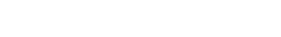B1
B1
B1
3

17
1. Mass after decrease
112 x 15/16
= 105 kg
Total decrease
(112 - 105) x 540
= 3780 kg
2.
1. No. of 90 kg bags
105 x 540
90
= 630
Least number of trips
630
120
= 5.25
→ 6 trips
2. Expenses
buying price = 1500 x 630
= 945000
transport = 2500 x 6
= 15000
Total 945000 + 15000
Selling price per bag:
= 960000 x 1.26
630
= 1920

M1
M1
A1

M1
M1
A1

M1
M1
A1
10

or equivalent
18
1. (x+3)(x - 2) = 24
x2 + x - 30 = 0
(x+6)(x - 5) = 0
x =-6 or x = 5
2.
1. (x + 9)x = 136
x2 + 9x – 136 = 0
(x + 17)(x - 9) = 0
x =-17 or x = 8
x = 8
perimeter
= 2(8 + 17) = 50 m
2. 2x × x = 136 - 64
2x2 = 72
x = 36
x = 6 m

M1
M1
A1

M1
M1
A1

B1
M1
A1

10

19
1. 2c + 9g = 98200
3c + 4g = 96000
2.cost of cow = sh 24800
cost of goat = sh 5400
3.
1. selling price of cows = 2 x 24800 x 1.3
selling price of goats = 9 x 5400 x 1.4
Total selling price
= 2 x 24800 x 1.3 + 9 x 5400 x 1.4
= 132520
2. % profit
132520 - 98200 x 100%
98200
= 34.95%

B1
B1
B1

M1
M1

A1

M1
A1

M1

A1

10

20
1.
1. Time taken by Juma = x/40h
Time taken by Mutuku = 80 - x
60
Let x km be distance from A
x  80 - x = ½
40       60
3x – 2(80 – x) = ½
120
2(5x – 160) = 120
10x = 440
x = 44 km
2. Time they met
10.00 am +44 h
40
= 10.00 +1 h 6 min
=11.06 am
2. Speed if Kamau delayed by 21 minutes
Kamau's time = ( 44 - 21)h
40   60
3/4h
speed needed:  44
3/4
= 58 2/3 km/h

B1
B1

M1
M1
A1

M1
A1

M1

M1

A1

10

21
1. Displacement, s, when t = 2
23 - 5 x 22 + 3 x 2 + 10
= 4
2.
1. velocity when t = 5 seconds
V = ds = 3t2 – 10t + 3
dt
when t = 5, V = 3 x 52 - 10 x 5+ 3
= 28
2. 3t2 - 10t + 3 = 0
(3t - 1)( -3) = 0
t =1/3 , = 3
3. time when velocity of particle is at its maximum
acceleration = dy = 61 - 10 = 0
t=10 =12/3s
6

M1
A1

B1

M1
A1

M1
M1
A1

M1
A1

10

22
1.
1. OB = p + q
=-p+3q
3. CB =-5q +p+q
= -4q +p
= k(-p+3q)
=- kq + 3kq
also
AX =-p+r(OB)
=-p+r(p + q)
= p(r - 1)+rq
p(r – 1)+ rq =-kp + 3kq
-k=r - 1 and r = 3k
-k = 3k-1
- 4k = -1 → k = ¼
substitute r = 3 x ¼ = 3/4

B1

M1

A1

M1
A1

B1

B1

M1

M1

A1

10

or equivalent
231. ABCD drawn
2.
1. Centre identified and used ✓
2. A"B"C"D"
3. A"B"C"D"
3. Reflection on line y =-x

B1

B1
B1
B1

B2
B2

B1
B1

10

AA', BB', CC' and DD' drawn
completion of square A'B'C'D' and labelled
A"B"C"D" drawn
A"B"C"D" drawn
reflection
line y=-x
24
1.
1. r/94/12
r = 9 x 4 = 3 cm
12
2. volume of material drilled out
1/3π x 3x 4
= 12π
2. Slart height of cone
= √92 + 122 = 15 cm
3. Surface area of solid after conical has been drilled
π x 9 x 15 + π x (92 - 32) + π x 3 x 5
=π(135 + 72 +15)
= 222π

M1

A1

M1
A1

B1

M1
M1
M1
M1
A1

10

for π x 9 x 15
for π (92 - 32)
π x 3 x 5 summing up

#### Download Kenya Certificate Of Secondary Education(KCSE 2013) Mathematics Alt A Paper 1 with Marking Scheme.

• ✔ To read offline at any time.
• ✔ To Print at your convenience
• ✔ Share Easily with Friends / Students